### Fibonacci Beginnings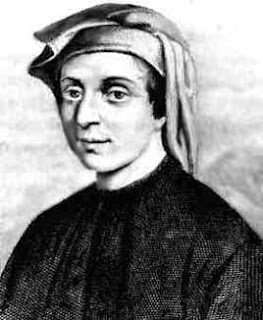In the early 1200’s, an Italian mathematician Leonardo of Pisa (nicknamed Fibonacci) discovered the famous Fibonacci sequence. This sequence falls under the Mathematical domain of number theory and its most famous problem concerned rabbits. The problem read:

Start with a single pair of rabbits. Any pair of rabbits of one generation will produce a pair for the next generation, and then another pair of rabbits for the generation after that. But then they will die. How many rabbits will be produced in the nth generation?

The answer to this well-known problem is the famed Fibonacci sequence: 1, 1, 2, 3, 5, 8, 13, 21…to get each subsequent term, add the sum of the two numbers that precede it. For example, 1+1=2= the third term in the sequence.

A fascinating extension to this sequence is that the Fibonacci numbers turn up in many areas of nature, as will be later discussed. Also, this sequence can be directly related to the Golden Ratio (1+√5/2 ) which results in the Golden Number Phi (1.618033988749…).

An amazing finding concerning the sequence is that the ratio of two consecutive Fibonacci numbers approaches the golden ratio or the golden number Phi. For example:
f(5)=5, f(4)=3
5/3 = 1.6666…
f(6)=8, f(5)=5
8/5=1.6
f(7)=13, f(6)=8
13/8=1.625
The values become closer and closer to the golden number as the sequence continues, which is a fascinating discovery.
It is also worthwhile to take note of the fact that if you multiply any term in the series by the value of the golden ratio, the answer that results will be close to the next number in the series. For example, 5 x 1.618=8.090. if higher numbers in the series are multiplied by the golden ratio, the result becomes closer and closers to the next number in the series.

Other than his great mathematical discoveries, there is very little known about Fibonacci’s personal life. However, these discoveries have made a tremendous impact on the mathematical world.

Resources:
http://www.lifeinitaly.com/heroes-villans/fibonacci.asp
http://www.mcs.surrey.ac.uk/Personal/R.Knott/Fibonacci/fibnat.html#golden
Mandl, M. (1984). Unusual Mathematical Puzzles, Tricks and Oddities. New Jersey: Prentice-Hall, Inc.

### Fibonacci in NatureThere are many areas of nature where the Fibonacci sequence can be found and some of these areas include, flower petals, plants, fruit, the human face, the human hand and animals (i.e. rabbits). The Fibonacci sequence can be found in the growth of living things ranging from simple cells to human beings (World Mysteries).

Flower petals follow the Fibonacci sequence and can identified in the growth of many plants. If you closely examine a flower that still has all of its petals intact, you will notice that the number of petals is a Fibonacci number (World Mysteries). For example, a calla lily has one petal, and a daisy can have twenty-one petals, with one and twenty-one being Fibonacci numbers. One of the most common flowers related to the Fibonacci sequence is the above mentioned daisy. Most field daisies consist of thirteen, twenty-one, thirty-four, fifty-five or eighty-nine petals, which are all numbers found in this famed sequence (World Mysteries). Other flowers whose number of petals is a Fibonacci number include lilies, euphorbia, trilliums, bloodroots, sunflowers, buttercups, and irises. The number of petals is not a product of chance, but rather a question of efficiency during the growth process of these flowers (World Mysteries).

The Fibonacci sequence can again be found in the natural surroundings and is part of the natural growth of many plants that are encountered everyday such as pinecones, fir cones, coniferous trees, and palm trees (World Mysteries).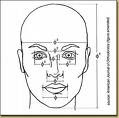The Fibonacci sequence has also been linked to the human face and hands. Many famous artists, for example, have used golden rectangles in the structure of their artwork. Of course, the concept of “golden rectangles and ratios” was noticed by Leonardo of Pisa (Fibonacci) as outlined in the above history of the Fibonacci sequence. Leonardo da Vinci’s painting of the Mona Lisa is based on the arrangement of the golden rectangle. Many argue that this is the reason behind its beauty.

The Fibonacci sequence can also be found in the structure of the human hand. Each part of the index finger, beginning from the tip down to the wrist, is larger than the preceding section by about the ratio of 1.618 (the golden ratio) (Human Hand).

This fascinating sequence is found in the beauty that is nature. Taking time out to appreciate the intricacies and subtleties of nature is sure to be an eye-opening and enlightening experience.

### Fibonacci in Nature: Fun Facts & Visuals

As mentioned, the Fibonacci sequence appears in many areas of nature. Fibonacci and the golden ratio can be found in:

Flower Petals

1 petal – white calla lily
2 petals - euphorbia
3 petals- iris & lily
5 petals - buttercup, wild rose, larkspur, columbine
8 petals - delphiniums
13 petals - ragwort, corn marigold, cineraria, black-eyed Susan
21 petals – Shasta daisy, aster, chicory
34 petals – field daisies, plantain, pyrethrum
55 or 89 petals - michelmas daisies, the asteraceae family

Fruits & Vegetables

A banana has 3 sections:

An apple has 5 sections:

Pineapple: The scales are patterned into spirals of hexagonal shapes of which three are distinct sets of spirals. One set of 5 spirals ascends at an angle to the right, a second set of 8 spirals rises steeply to the left and the third set of 13 spirals rises very steeply to the right.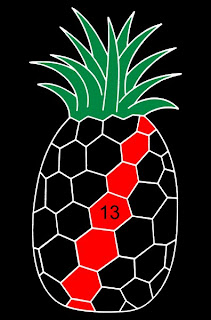A cauliflower also shows the golden spiral.

Animals

Dolphins: The eyes, fins and tail of the dolphin are golden numbers and the fins of a dolphin are divided using the ratio as well.

Penguin: The eyes, beak, wing and certain body markings of the penguin all fall at golden sections of its height.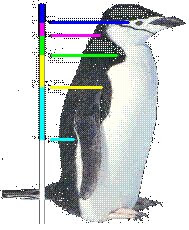Human Body

The ear reflects the shape of the fibonacci spiral and the cochlea of our inner ear forms a golden spiral as well.

Fingers: 8 fingers in total, 5 digits on each hand, 3 bones in each finger, 2 bones in 1 thumb, and 1 thumb on each hand.

Music

A piano keyboard has 8 white keys, 5 black keys in groups of 2 and 3These 13 keys comprise one octave.

### Fibonacci in the Classroom

The Fibonacci sequence can be used in many different subject areas and it can be used to teach patterns in math, music, art, language arts and science. The teaching of patterns begins in the early grades where students are required to identify various forms of patterning and continues into the elementary grades. For example, an elementary mathematics course outcome for the Newfoundland and Labrador curriculum is that students will explore, recognize, represent and apply patterns and relationships both formally and informally. When teaching mathematical patterns, teachers can relate the Fibonacci sequence to patterns that can be seen in real life such as those of flower petals.

The sequence can be taught within the music curriculum using the keys on a piano, which as mentioned above, follow the Fibonacci number pattern. Teachers can demonstrate to the students that the piano follows the Fibonacci sequence by having 5 black keys, 8 white keys and 13 notes which comprise one octave. This sequence can also be identified in various music scales such as the pentatonic, diatonic, and the chromatic scales (Math and Music).

The Fibonacci sequence can also be found in the area of science and more specifically, in the areas of space and physics. When teaching a unit on space, teachers can provide children with interesting facts on how the universe is based on the Golden Ratio, as there are many galaxies in the universe that contain the Golden Ratio in their structure (Measure of Beauty). Also, teachers can use the Fibonacci sequence when teaching a unit on light. For example, when light is held over a surface (i.e. glass), one part of the light passes through the surface, one part is absorbed and one part is reflected. Therefore a multiple reflection occurs which means that there are a number of reflections that the ray of light takes inside the glass before it re-emerges. The number of rays that re-emerge reflect the Fibonacci number sequence (Measure of Beauty).

Within the language arts curriculum there are ways to bring in the concept of the Fibonacci sequence and its numbers. For example, in the area of poetry, several of the rhythms used are Fibonacci numbers, such as iambic pentameter. As well, the number of lines in particular forms of poetry are Fibonacci numbers, such as limericks, which have five lines or Haiku poems which have three.

The Fibonacci sequence can also be used in teaching spatial proportions in art education. Teachers can use spirals, concentric circles and repeated rectangles to show how interesting patterns can be formed using these figures when teaching students about proportional preferences (The Fibonacci Sequence, p. 92). Also, when teaching a section on portrait drawing, it is important to inform students of the correct facial and body proportions when drawing a human figure. These proportions enable the students to create a drawing that is lifelike and directly proportional to the subject he/she is drawing.

The Fibonacci sequence does not have to be strictly limited to the mathematics curriculum. Incorporating it into other subject areas will allow students to see how the sequence can be relevant to "real life" which makes the information meaningful and relevant for the student. Above all else, this should be a primary goal of any mathematics curriculum.

### Lesson Plans

Here are some links to lesson plans that teachers can use when teaching the Fibonacci sequence and the golden ratio.

Fibonacci Number Sequence and Wild Flowers
This lesson plan requires students to explore and apply the Fibonacci sequence to solve problems and examine how it relates to the environment.

Math and Music
This lesson plan requires students to describe the Fibonacci sequence and explore the relationship between math and music.

Earth Art: Creating Patterns in Nature
This lesson plan requires students to explore the artwork of Goldsworthy and Fibonacci. It provides an excellent extension activity that requires children to create their own artwork based on the Fibonacci sequence.

The Fibonacci Sequence
This lesson plan requires children to investigate numerical patterns in mathematics and to look for evidence of numerical patterns in nature.

The Golden Ratio
This lesson requires students to use various materials to create rectangles based on the golden ratio to see how it was used in ancient architecture.

Fibonacci Numbers and the Golden Section
This lesson plan enables children to understand the Fibonacci sequence and the Golden Section.

Classroom Activities Using Number Patterns
This lesson plan requires students to form sequences and patterns to figure out the Fibonacci rule.

Fun with Fibonacci
This website contains various lesson plans that teachers can use to teach number patterns and sequences using the Fibonacci generalization. It also provides a section of mini-lessons to help them complete the lesson.

### Fibonacci Books for the Classroom

In addition to the information below, further information on the following books can be found on http://www.amazon.ca/.Fibonacci Fun by Trudi Hammel Garland
ISBN: 1572322659

From "Raising Rabbits" to "Prickly Pinecones", 24 easy-to-use, reproducible activities and projects introduce students to Fibonacci numbers and the golden ratio. Grades 4-8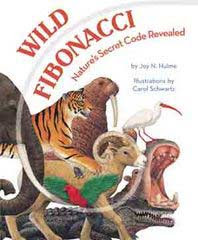Wild Fibonacci: Nature's Secret Code Revealed by Joy N. Hulme and Carol Schwartz
ISBN:
1582461546

In Wild Fibonacci, readers will discover this mysterious code in a special shape called an equiangular spiral. Joy Hulme provides a clear and accessible introduction to the Fibonacci sequence and its presence in the animal world.

ISBN: 1570918953

A Fibonacci Tale - Math Adventure. It is up to a young girl named Amanda to save Chee crops by figuring out the pattern by which the rabbits multiply.Fascinating Fibonaccis: Mystery and Magic in Numbers by Trudi Hammel Garland
ISBN: 0866513434

Explore the Fibonacci numbers, ratios and occurrences in natural phenomena.

### It's Fibonacci Fun Time!

Fun Links to Games and Activities!
This website is an excellent interactive informational source for children. It provides videos, quizzes, an “ask us” section, and related topics to Fibonacci.
This webquest is an excellent site that allows children to explore the Fibonacci sequence with bunnies, bees, and plants!
This website provides various puzzles that allows children to see explanations to why the answers involve the Fibonacci sequence.
This site provides quizzes for children to complete in which they have to understand the Fibonacci sequence.
This site is an excellent site for children to construct the golden spiral using squares that represent the Fibonacci sequence. Children are required to assemble various squares in the correct sequence to create the golden spiral.

### Fibonacci Reflections...

When I began this inquiry project, I originally wanted to learn about the Fibonacci sequence itself, and why it is such a famous sequence. Once I began the research, I quickly discovered the answer to this question. This sequence has intricacies and subtleties that I never realized existed. I had heard about the Golden Ratio, but I also didn't realize how closely it was linked with the famed Fibonacci sequence. This discovery has made this inquiry a highly worthwhile endeavour.

I wondered how I could teach my students about this sequence in such a way that focused on a problem-solving approach. I didn't want to just tell them "here's the Fibonacci sequence. The numbers in this sequence are 1, 1, 2, 3... and you get each number by adding the two preceding terms". This is a didactic approach and one that will not motivate students to want to learn about the Fibonacci sequence. They will memorize the formula and that will be the end of it for them--in one ear and out the other. The fact that the Fibonacci sequence is so closely related with the Golden Ratio opened up a whole new world of Fibonacci possibilities. Students have tangible examples at their fingertips to see that the Fibonacci sequence has a tremendous impact on many areas of nature, on human and animal body proportions and in many other areas as well.

Because the sequence is so prevalent in many areas that are familiar to students, they will become motivated to learn about this topic and therefore, the knowledge that they acquire will be meaningful to them and processed at a much deeper level.

The great thing about this topic is that students will actually be able to see for themselves the presence of the Fibonacci numbers and the golden ratio. They can measure and observe people and objects around them to find new areas on their own where this sequence can be found. The benefits of having students construct some of their own knowledge about this topic allows for a deeper understanding of it. This constructivist approach is a highly effective way to teach mathematics. As well, adopting a problem-solving approach makes instruction much more effective. Students have to be taught to think logically and critically and a way that this can be accomplished is to let them practice with problem solving approaches that will foster this growth. All areas and topics in a mathematics curriculum should be taught with these philosophies in mind. The end result will be students who are confident and motivated to learn, and this should be of utmost consideration when developing lessons for any topic in a mathematics curriculum.

### Resources Used

Fibonacci Numbers and Nature
http://www.mcs.surrey.ac.uk/Personal/R.Knott/Fibonacci/fibnat.html

World Mysteries
http://www.world-mysteries.com/sci_17.htm

Fibonacci in Nature
http://jwilson.coe.uga.edu/EMAT6680/Parveen/Fib_nature.htm

Math and Music
http://www.teachervision.fen.com/math-principles/lesson-plan/10320.html

Plants
http://evolutionoftruth.com/goldensection/plants.htm

Nature
http://techcenter.davidson.k12.nc.us/Group2/nature.htm

The Human Face
http://goldennumber.net/face.htm

Animals
http://goldennumber.net/nature2.htm

Fibonacci Numbers in Nature
http://britton.disted.camosun.bc.ca/fibslide/jbfibslide.htm

A Measure of Beauty
http://www.islamonline.net/English/Science/2005/03/article01.shtml

Keyboard Picture
http://www.hausarbeiten.de/faecher/hausarbeit/ing/24705.html

Devlin, K. (2002). Numbers in the Garden and Geometry in the Jungle. Mathematics Teaching in the Middle School, 7(8), 422-425.

Mandl, M. (1984). Unusual Mathematical Puzzles, Tricks and Oddities. New Jersey: Prentice- Hall, Inc.

Miller, C. B. & Veenestra, Tamara B. (2002). Fibonacci: Beautiful Patterns, Beautiful Numbers. Mathematics Teaching in the Middle School, 7(5), 298-305.

Neperud, R. W. & Serlin, R. C. (1984). The Fibonacci Sequence: Proportional and Semantic Bases of Children's Aesthetic Preferences. Art Education, 25(2), 92-103.

Newfoundland and Labrador Mathematics Curriculum Documents
http://www.ed.gov.nl.ca/edu/sp/mathlist.htm